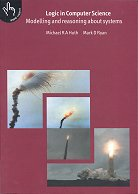## Books : reviews### Michael R. A. Huth, Mark D. Ryan.Logic in Computer Science: modelling and reasoning about systems. CUP. 2000

rating : 3 : worth reading
review : 4 October 2000

Most books on logic are aimed at pure mathematicians. Here is a lovely overview of lots of "applied logics", directed at computer scientists. It covers a range of uses of logic: propositional and predicate logics, conventional program proving, engineering a modal logic for your particular application, and model checking.

Everything is motivated by good introductory sections and good examples, before leaping into the symbols. One nice theme running through the book is that of comparing and contrasting doing proofs by using inference rules, and finding meanings by calculating the semantics.

Given the breadth, nothing is covered in sufficient depth to be able to go out and start using the techniques in anger. But it gives a solid grounding, ready for the next stage of learning, and the uniform treatment of such a wide range of logic applications gives a wonderful feel for the underlying unity of the subject.

Recommended.

#### Contents:

1. Propositional logic: proofs by natural deduction; induction; semantics by truth tables; canonical normal forms
2. Predicate logic: its proof theory and semantics; undecidability
3. Verification by model checking: CTL (computation tree logic); model checking algorithms; SMV (Symbolic Model Verifier); fairness; LTL (linear-time temporal logic), CTL*; fixed points
4. Program verification: Hoare triples; Floyd-Hoare style proof calculus; partial and total correctness
5. Modal logics and agents: modes of truth; logic engineering; reasoning about knowledge; multi-agent systems
6. Binary Decision Diagrams (BDDs): boolean functions; Ordered BDDs (OBDDs); algorithms for reduced OBDDs; symbolic model checking; a relational mu-calculus